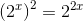# ISEE Upper Level Quantitative : How to find the area of a square

## Example Questions

← Previous 1

### Example Question #10 : Squares

The perimeter of a square is one yard. Which is the greater quantity?

(a) The area of the square

(b)square foot

(a) and (b) are equal.

It is impossible to tell form the information given.

(a) is greater.

(b) is greater.

(a) is greater.

Explanation:

One yard is equal to three feet, so the length of one side of a square with this perimeter isfeet. The area of the square is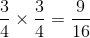square feet.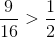, making (a) greater.

### Example Question #1 : How To Find The Area Of A Square

Square 1 is inscribed inside a circle. The circle is inscribed inside Square 2.

Which is the greater quantity?

(a) Twice the area of Square 1

(b) The area of Square 2

(a) is greater.

(b) is greater.

(a) and (b) are equal.

It is impossible to tell from the information given.

(a) and (b) are equal.

Explanation:

Letbe the sidelength of Square 1. Then the length of a diagonal of this square - which istimes this sidelength, or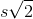, by the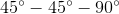Theorem - is the same as the diameter of this circle, which, in turn, is equal to the sidelength of Square 2.

Therefore, Square 1 has area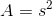, and Square 2 has area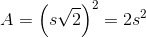, twice that of Square 1.

### Example Question #2 : How To Find The Area Of A Square

Which is the greater quantity?

(A) The area of a square with sidelength one foot

(B) The area of a rectangle with length nine inches and height fourteen inches

(A) and (B) are equal

(A) is greater

It is impossible to determine which is greater from the information given

(B) is greater

(A) is greater

Explanation:

The area of a square is the square of its sidelength, which here is 12 inches: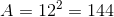square inches.

The area of a rectangle is its length multiplied by its height, which, respectively, are 9 inches and 14 inches: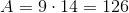square inches.

The square has the greater area.

### Example Question #3 : How To Find The Area Of A Square

A square lawn has sidelength twenty yards. Give its area in square feet.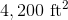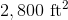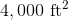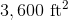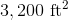Explanation:

20 yards converts to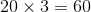feet. The area of a square is the square of its sidelength, so the area in square feet is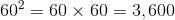square feet.

### Example Question #4 : How To Find The Area Of A Square

Rectangle A and Square B both have perimeter 2 meters. Rectangle A has width 25 centimeters. The area of Rectangle A is what percent of the area of Square B?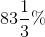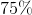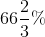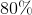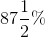Explanation:

The perimeter of a rectangle can be given by the formula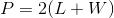Rectangle A has perimeter 2 meters, which is equal to 200 centimeters, and width 25 centimeters, so the length is: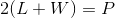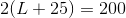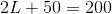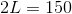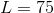The dimensions of Rectangle A are 75 centimeters and 25 centimeters, so its area is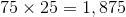square centimeters.

The sidelength of a square is one-fourth its perimeter, which here is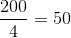centimeters; its area is therefore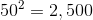square centimeters.

The area of Rectangle A is therefore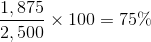that of Square B.

### Example Question #5 : How To Find The Area Of A Square

The sidelength of Square A is three-sevenths that of Square B. What is the ratio of the area of Square B to that of Square A?

None of the other answers give the correct ratio.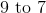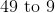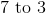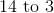Explanation:

Since the ratio is the same regardless of the sidelengths, then for simplicity's sake, assume the sidelength of Square B is 7. The area of Square B is therefore the square of this, or 49.

Then the sidelength of Square A is three-sevenths of 7, or 3. Its area is the square of 3, or 9.

The ratio of the area of Square B to that of Square A is therefore 49 to 9.

### Example Question #6 : How To Find The Area Of A Square

Five squares have sidelengths one foot, two feet, three feet, four feet, and five feet.

Which is the greater quantity?

(A) The mean of their areas

(B) The median of their areas

It is impossible to tell which is greater from the information given

(A) and (B) are equal

(A) is greater

(B) is greater

(A) is greater

Explanation:

The areas of the squares are: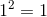square foot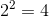square feet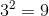square feet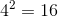square feet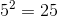square feet

Therefore, we are comparing the mean and the median of the set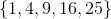.

The mean of this set is the sum divided by 5: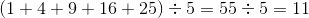The median is the middle element after arrangement in ascending order, which is 9.

This makes (A), the mean, greater.

### Example Question #7 : How To Find The Area Of A Square

Four squares have sidelengths one meter, 120 centimeters, 140 centimeters, and 140 centimeters. Which is the greater quantity?

(A) The mean of their areas

(B) The median of their areas

(A) is greater

(A) and (B) are equal

It is impossible to tell which is greater from the information given

(B) is greater

(B) is greater

Explanation:

The areas of the squares are: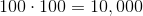square centimeters (one meter being 100 centimeters)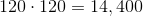square centimeters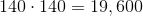square centimeterssquare centimeters

The mean of these four areas is their sum divided by four: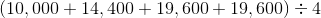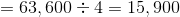square centimeters.

The median is the mean of the two middle values, or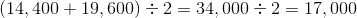square centimeters.

The median, (B), is greater.

### Example Question #8 : How To Find The Area Of A Square

The perimeter of a square is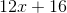. Give the area of the square in terms of.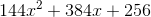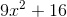None of the other responses gives a correct answer.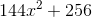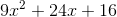Explanation:

The length of one side of a square is one fourth its perimeter. Since the perimeter of the square is, the length of one side is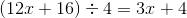The area of the square is the square of this sidelength, or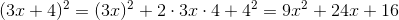### Example Question #9 : How To Find The Area Of A Square

The sidelength of a square is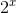. Give its area in terms of.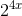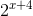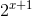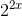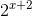The area of a square is the square of its sidelength. Therefore, square: